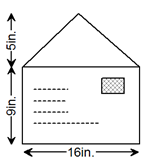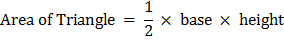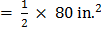### Sample Problem

What is the area of the envelope (including the stamp area) ?The area of the envelope is in.2.

#### Solution

Solution:

Area of rectangle = base × height

= 16 in. × 9 in.

= 144 in.2.== 40 in.2

Area of the envelope = Area of rectangle + Area of triangle ABE

= 144 in.2 + 40 in.2

The area of the envelope is 184 in.2